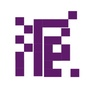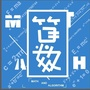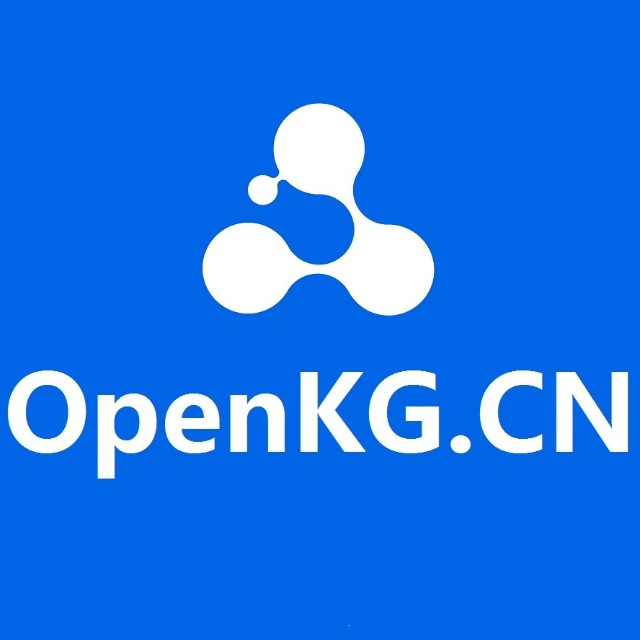We consider the problem of zero-shot recognition: learning a visual classifier for a category with zero training examples, just using the word embedding of the category and its relationship to other categories, which visual data are provided. The key to dealing with the unfamiliar or novel category is to transfer knowledge obtained from familiar classes to describe the unfamiliar class. In this paper, we build upon the recently introduced Graph Convolutional Network (GCN) and propose an approach that uses both semantic embeddings and the categorical relationships to predict the classifiers. Given a learned knowledge graph (KG), our approach takes as input semantic embeddings for each node (representing visual category). After a series of graph convolutions, we predict the visual classifier for each category. During training, the visual classifiers for a few categories are given to learn the GCN parameters. At test time, these filters are used to predict the visual classifiers of unseen categories. We show that our approach is robust to noise in the KG. More importantly, our approach provides significant improvement in performance compared to the current state-of-the-art results (from 2 ~ 3% on some metrics to whopping 20% on a few).0+10+下载关闭预览

### 更多0+12+0+0+5+0+0+4+0+1+6+0+0+2+0+The potential of graph convolutional neural networks for the task of zero-shot learning has been demonstrated recently. These models are highly sample efficient as related concepts in the graph structure share statistical strength allowing generalization to new classes when faced with a lack of data. However, knowledge from distant nodes can get diluted when propagating through intermediate nodes, because current approaches to zero-shot learning use graph propagation schemes that perform Laplacian smoothing at each layer. We show that extensive smoothing does not help the task of regressing classifier weights in zero-shot learning. In order to still incorporate information from distant nodes and utilize the graph structure, we propose an Attentive Dense Graph Propagation Module (ADGPM). ADGPM allows us to exploit the hierarchical graph structure of the knowledge graph through additional connections. These connections are added based on a node's relationship to its ancestors and descendants and an attention scheme is further used to weigh their contribution depending on the distance to the node. Finally, we illustrate that finetuning of the feature representation after training the ADGPM leads to considerable improvements. Our method achieves competitive results, outperforming previous zero-shot learning approaches.0+10+0+下载预览0+3+0+0+12+0+0+19+0+
Top